# Directed Graphical Probabilistic Models learning in DGMs William

• Slides: 71Directed Graphical Probabilistic Models: learning in DGMs William W. Cohen Machine Learning 10 -601 Slide 1REVIEW OF DGMS Slide 2Another difficult problem: common-sense reasoning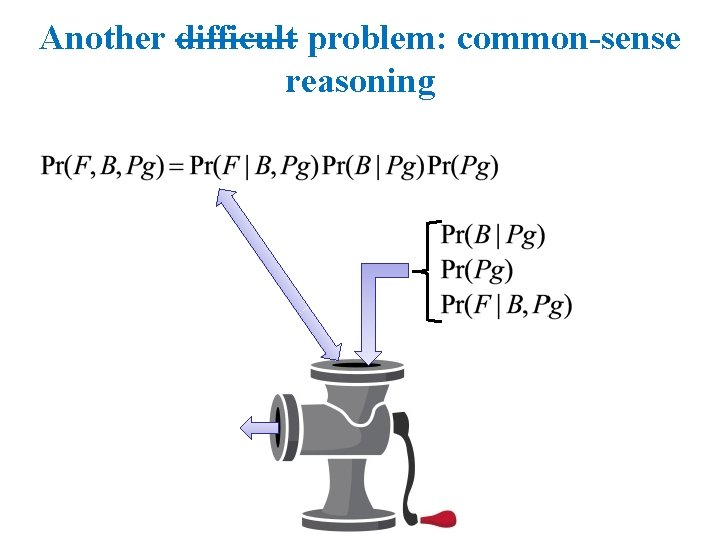Another difficult problem: common-sense reasoningSummary of Monday(1): Bayes nets • • • Many problems can be solved using the joint probability P(X 1, …, Xn). Bayes nets describe a way to compactly write the joint. For a Bayes net: A P(A) 1 0. 33 2 0. 33 3 0. 33 First guess The money A B Stick or swap? Second guess • Conditional independence: E A C D P(E|A, C, D) … … P(B) 1 0. 33 2 0. 33 3 0. 33 The goat C D B A B C P(C|A, B) 1 1 2 0. 5 1 1 3 0. 5 1 2 3 1. 0 1 3 2 1. 0 … … Slide 5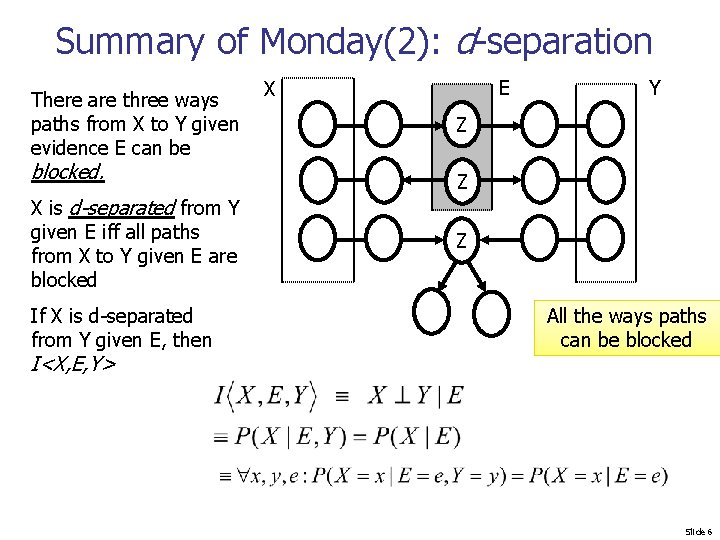Summary of Monday(2): d-separation There are three ways paths from X to Y given evidence E can be blocked. X is d-separated from Y given E iff all paths from X to Y given E are blocked If X is d-separated from Y given E, then I<X, E, Y> E X Y Z Z Z All the ways paths can be blocked Slide 6Quiz 1: which are d-separated? Evidence nodes are filled X 1 Z Y 1 X 2 Z Y 2 X 3 Z Y 3 https: //piazza. com/class/ij 382 zqa 2572 hc? cid=444 Slide 7Quiz 2: which is an example of explaining away? Evidence nodes are filled X 2 Z Y 2 X 3 Z Y 3 https: //piazza. com/class/ij 382 zqa 2572 hc? cid=445 Slide 8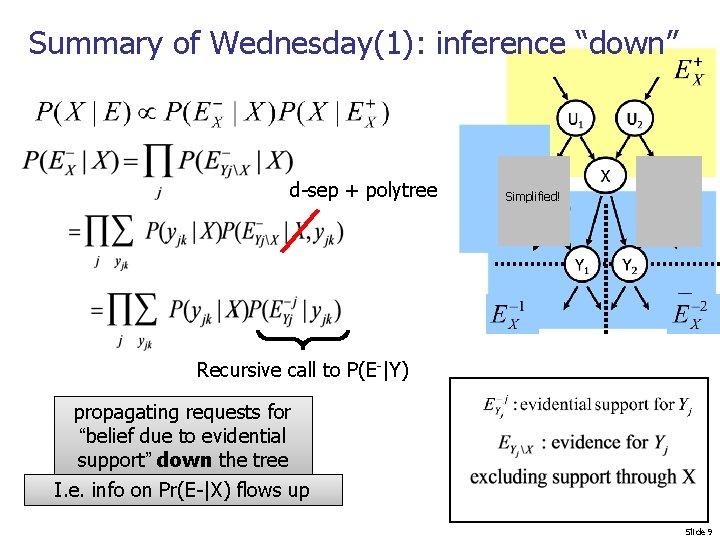Summary of Wednesday(1): inference “down” d-sep + polytree Simplified! Recursive call to P(E-|Y) propagating requests for “belief due to evidential support” down the tree I. e. info on Pr(E-|X) flows up Slide 9Summary of Wed(2): inference “up” CPT table lookup Recursive call to P(. |E+) Evidence for Uj that doesn’t go thru X propagating requests for “belief due to causal evidence” up the tree I. e. info on Pr(X|E+) flows down Slide 10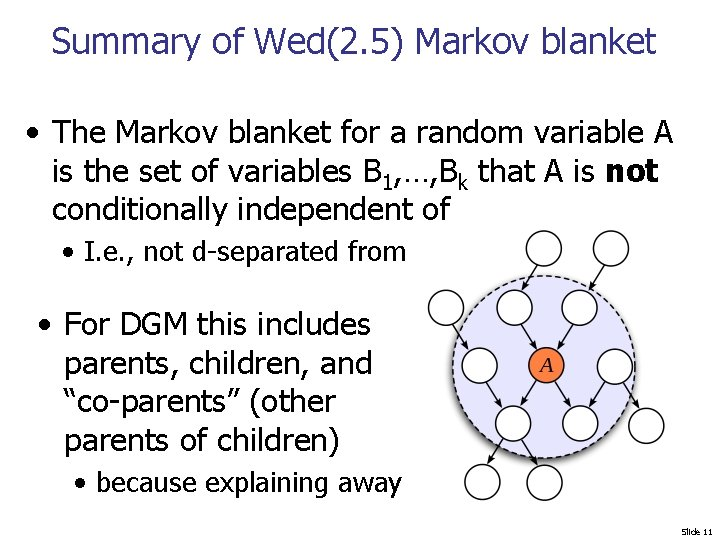Summary of Wed(2. 5) Markov blanket • The Markov blanket for a random variable A is the set of variables B 1, …, Bk that A is not conditionally independent of • I. e. , not d-separated from • For DGM this includes parents, children, and “co-parents” (other parents of children) • because explaining away Slide 11Summary of Wed(3): sideways? ! recursion Similar ideas as before but more complex recursion: • Z’s are NOT independent of X (because explaining away) • Neither are they strictly higher or lower in the tree. Slide 12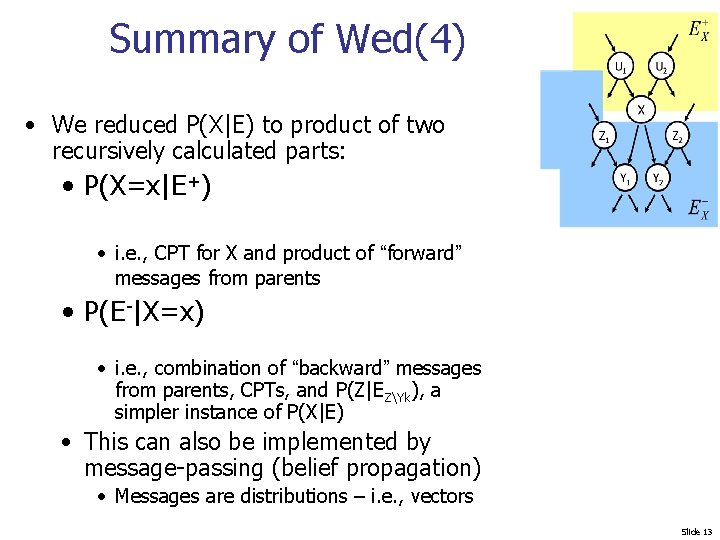Summary of Wed(4) • We reduced P(X|E) to product of two recursively calculated parts: • P(X=x|E+) • i. e. , CPT for X and product of “forward” messages from parents • P(E-|X=x) • i. e. , combination of “backward” messages from parents, CPTs, and P(Z|EZYk), a simpler instance of P(X|E) • This can also be implemented by message-passing (belief propagation) • Messages are distributions – i. e. , vectors Slide 13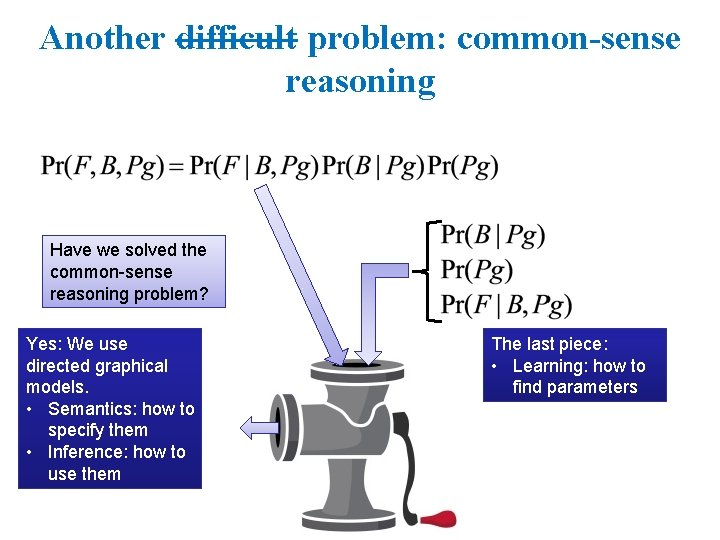Another difficult problem: common-sense reasoning Have we solved the common-sense reasoning problem? Yes: We use directed graphical models. • Semantics: how to specify them • Inference: how to use them The last piece: • Learning: how to find parametersLEARNING IN DGMSLearning for Bayes nets • Input: - Sample of the joint: - Graph structure of the variables • for I=1, …, N, you know Xi and parents(Xi) A • Output: - Estimated CPTs Method (discrete variables): B C D • Estimate each CPT independently E • Use a MLE or MAP … B P(B) 1 0. 33 2 0. 33 3 0. 33 A B C P(C|A, B) 1 1 2 0. 5 1 1 3 0. 5 1 2 3 1. 0 1 3 2 1. 0 … …Learning for Bayes nets Method (discrete variables): • • Estimate each CPT independently A Use a MLE or MAP estimate for B C D • MLE estimate: E … B P(B) 1 0. 33 2 0. 33 3 0. 33 A B C P(C|A, B) 1 1 2 0. 5 1 1 3 0. 5 1 2 3 1. 0 1 3 2 1. 0 … …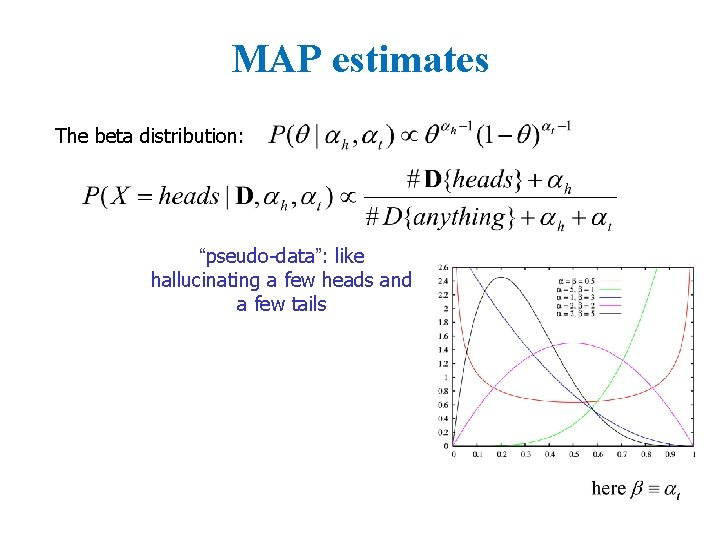MAP estimates The beta distribution: “pseudo-data”: like hallucinating a few heads and a few tails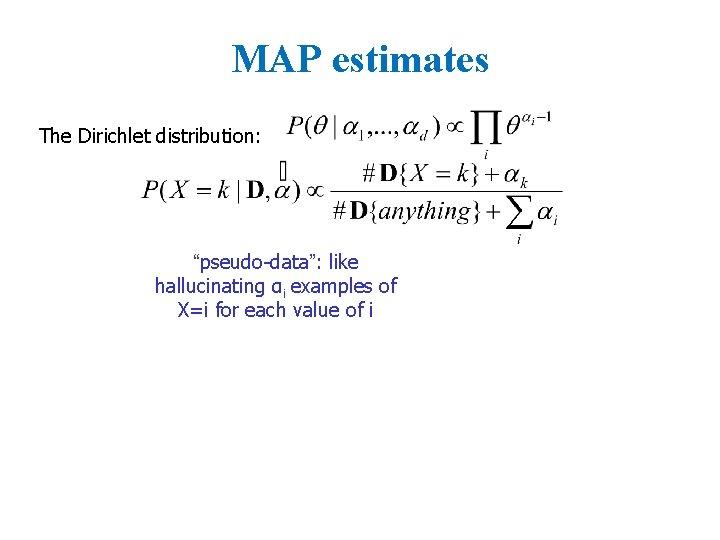MAP estimates The Dirichlet distribution: “pseudo-data”: like hallucinating αi examples of X=i for each value of i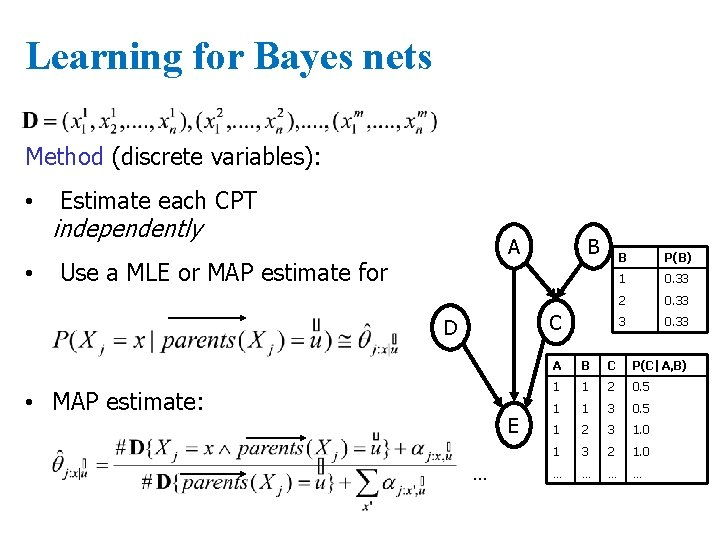Learning for Bayes nets Method (discrete variables): • • Estimate each CPT independently A Use a MLE or MAP estimate for B C D • MAP estimate: E … B P(B) 1 0. 33 2 0. 33 3 0. 33 A B C P(C|A, B) 1 1 2 0. 5 1 1 3 0. 5 1 2 3 1. 0 1 3 2 1. 0 … …Learning for Bayes nets Method (discrete variables): • • Estimate each CPT independently A Use a MLE or MAP estimate for C D • MAP estimate: B E B P(B) 1 0. 33 2 0. 33 3 0. 33 A B C P(C|A, B) 1 1 2 0. 5 1 1 3 0. 5 1 2 3 1. 0 1 3 2 1. 0 … …WAIT, THAT’S IT?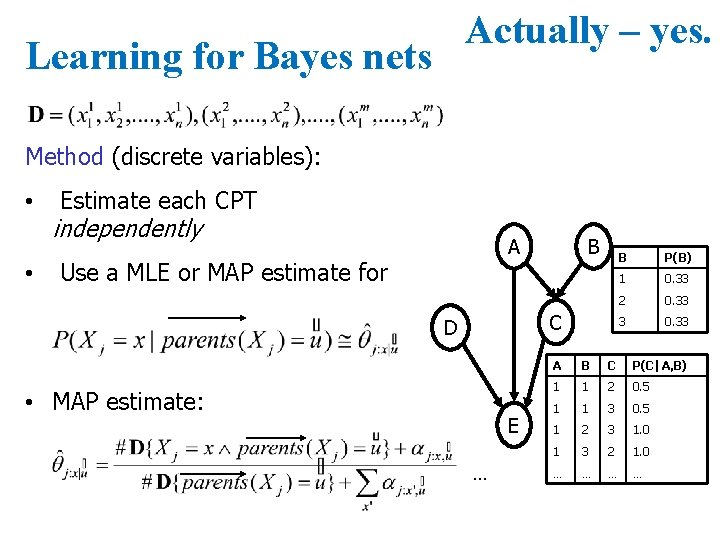Actually – yes. Learning for Bayes nets Method (discrete variables): • • Estimate each CPT independently A Use a MLE or MAP estimate for B C D • MAP estimate: E … B P(B) 1 0. 33 2 0. 33 3 0. 33 A B C P(C|A, B) 1 1 2 0. 5 1 1 3 0. 5 1 2 3 1. 0 1 3 2 1. 0 … …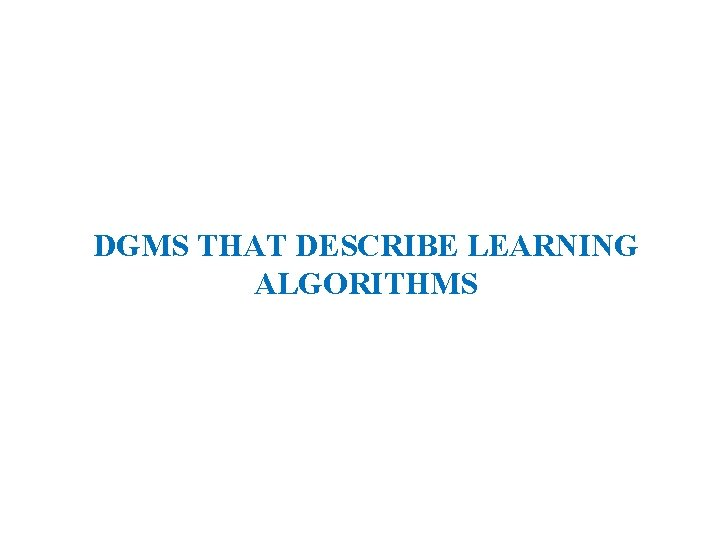DGMS THAT DESCRIBE LEARNING ALGORITHMS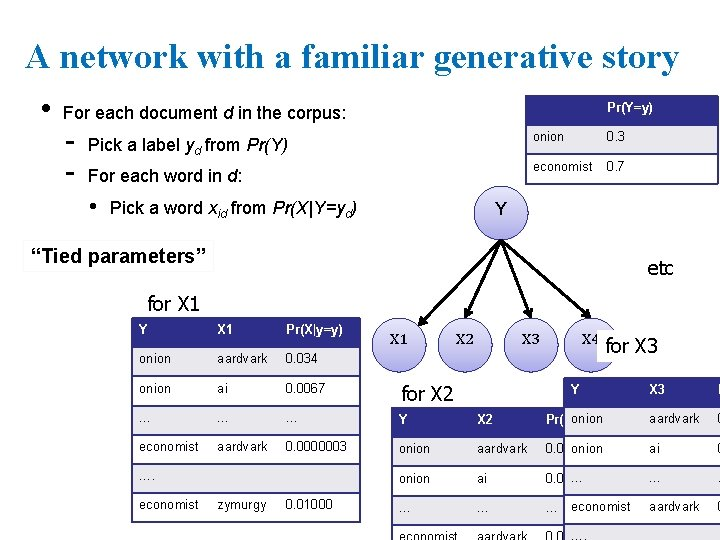A network with a familiar generative story • Pr(Y=y) For each document d in the corpus: - Pick a label yd from Pr(Y) For each word in d: • Pick a word xid from Pr(X|Y=yd) onion 0. 3 economist 0. 7 Y “Tied parameters” etc for X 1 Y X 1 Pr(X|y=y) onion aardvark 0. 034 onion ai 0. 0067 for X 2 … … … Y X 2 economist aardvark 0. 0000003 onion …. economist zymurgy 0. 01000 X 1 X 2 X 3 X 4 for X 3 Y X 3 P onion Pr(X|y=y) aardvark 0 aardvark onion 0. 034 ai 0 onion ai … 0. 0067 … … … economist aardvark 0A network with a familiar generative story • Pr(Y=y) For each document d in the corpus (of size D): - Pick a label yd from Pr(Y) For each word in d (of length Nd): • onion 0. 3 economist 0. 7 Pick a word xid from Pr(X|Y=yd) Y for every X Y X Pr(X|y=y) onion aardvark 0. 034 onion ai 0. 0067 … … … economist aardvark 0. 0000003 …. economist X Nd D zymurgy 0. 01000 Plate diagram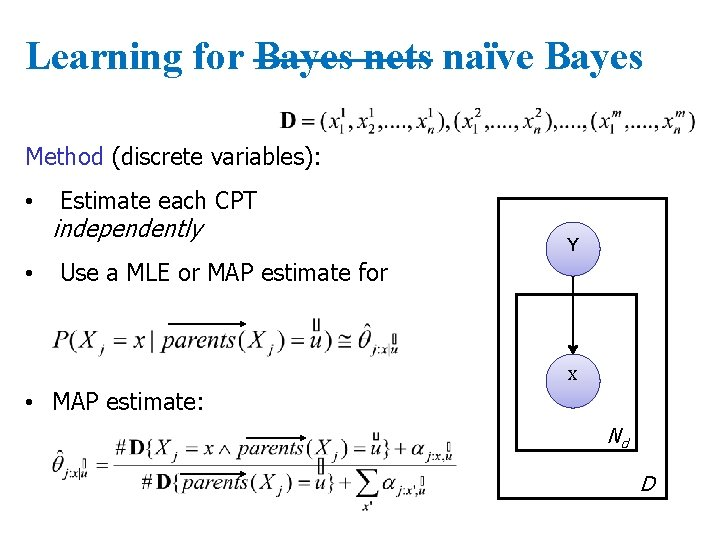Learning for Bayes nets naïve Bayes Method (discrete variables): • • Estimate each CPT independently Y Use a MLE or MAP estimate for X • MAP estimate: Nd D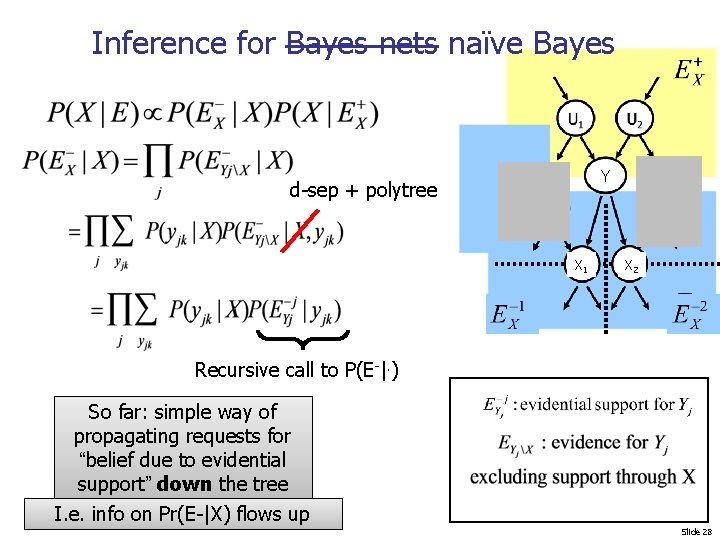Inference for Bayes nets naïve Bayes Y d-sep + polytree X 1 X 2 Recursive call to P(E-|. ) So far: simple way of propagating requests for “belief due to evidential support” down the tree I. e. info on Pr(E-|X) flows up Slide 28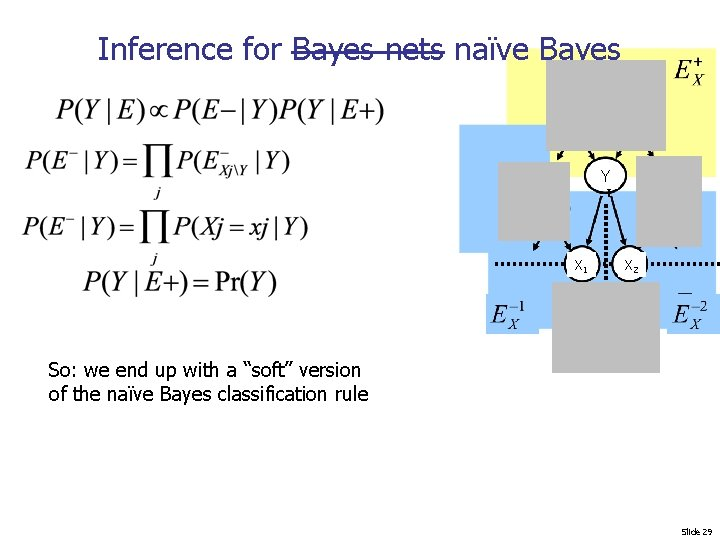Inference for Bayes nets naïve Bayes Y Y X 1 X 2 So: we end up with a “soft” version of the naïve Bayes classification rule Slide 29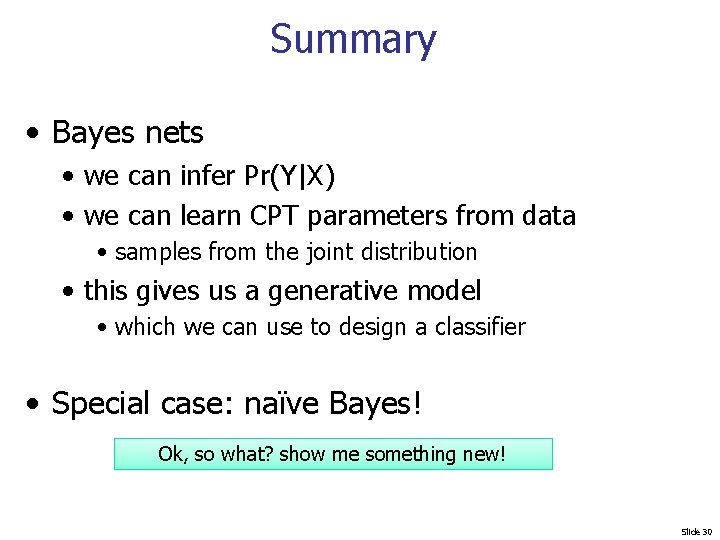Summary • Bayes nets • we can infer Pr(Y|X) • we can learn CPT parameters from data • samples from the joint distribution • this gives us a generative model • which we can use to design a classifier • Special case: naïve Bayes! Ok, so what? show me something new! Slide 30LEARNING IN DGMS I lied, there’s actually more. Hidden (or latent) variables Slide 31• If we know the parameters (the CPTs) I can infer the values of the hidden variables. • But, we don’t • If we know the values of the hidden variables we can learn the values of the parameters (the CPTs) • But, we don’t Hidden (or latent) variables Slide 32Learning with Hidden Variables Hidden variables: what if some of your data is not completely observed? Method: Z X Y Expectation-maximization aka EM Z X Y ugrad <20 facebook ugrad 20 s facebook grad 20 s thesis weighting each example by confidence in its correctness. expectation grad 20 s facebook prof 30+ grants 4. Re-estimate parameters using the extended dataset (real + pseudo-data). ? <20 facebook ? 30 s thesis 5. Repeat starting at step 2…. 1. Estimate parameters θsomehow or other. 2. Predict values of hidden variables using θ. 3. Add pseudo-data corresponding to these predictions, maximization (MLE/MAP) Slide 33Learning with Hidden Variables: Example Z X 1 X 2 ugrad <20 facebook ugrad 20 s facebook grad 20 s thesis grad 20 s facebook prof 30+ grants Z X 1 P(X 1|Z) Z X 2 P(X 2|Z) ? <20 facebook undergr <20 . 4 undergr facebk . 6 ? 30 s thesis 20 s . 4 thesis . 2 30+ . 2 grants . 2 <20 . 2 facebk . 4 20 s . 6 thesis . 4 30+ . 2 grants . 2 <20 . 25 facebk . 25 20 s . 25 thesis . 25 30+ . 5 grants . 5 grad prof Z P(Z) undergr 0. 333 grad 0. 333 prof 0. 333 grad prof Z X 1 X 2 Slide 34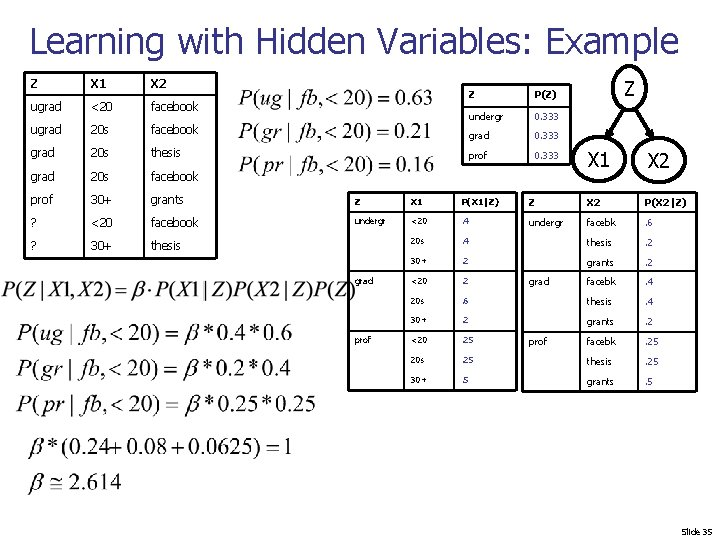Learning with Hidden Variables: Example Z X 1 X 2 ugrad <20 facebook ugrad 20 s facebook grad 20 s thesis grad 20 s facebook prof 30+ grants Z X 1 P(X 1|Z) ? <20 facebook undergr <20 . 4 ? 30+ thesis 20 s grad prof Z P(Z) undergr 0. 333 grad 0. 333 prof 0. 333 Z X 1 X 2 Z X 2 P(X 2|Z) undergr facebk . 6 . 4 thesis . 2 30+ . 2 grants . 2 <20 . 2 facebk . 4 20 s . 6 thesis . 4 30+ . 2 grants . 2 <20 . 25 facebk . 25 20 s . 25 thesis . 25 30+ . 5 grants . 5 grad prof Slide 35Learning with Hidden Variables: Example Z X 1 X 2 ugrad <20 facebook ugrad 20 s facebook grad 20 s thesis grad 20 s facebook prof 30+ grants Z X 1 ugrad <20 facebook undergr <20 grad <20 facebook prof <20 facebook ugrad 30+ thesis prof 30+ thesis Z Z P(Z) undergr grad X 1 X 2 Z X 2 P(X 2|Z) undergr facebk prof grad prof P(X 1|Z) 20 s thesis 30+ grants <20 grad facebk 20 s thesis 30+ grants <20 prof facebk 20 s thesis 30+ grants Slide 37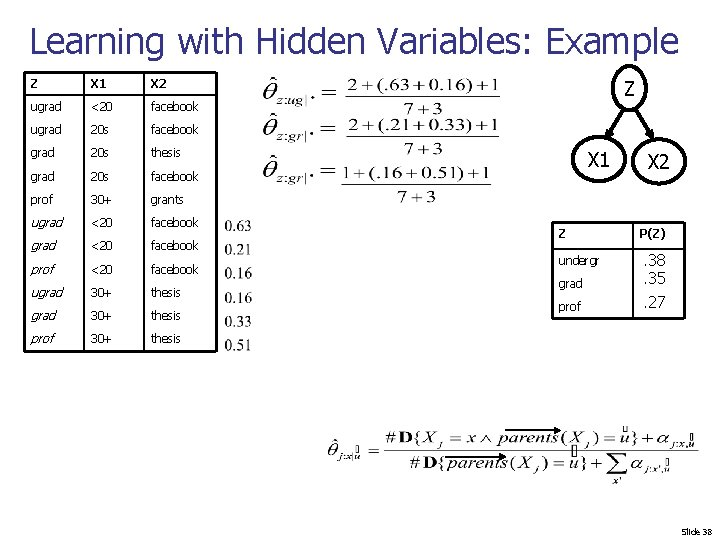Learning with hidden variables Hidden variables: what if some of your data is not completely observed? Z X 1 X 2 Method: 1. Estimate parameters somehow or other. 2. Predict unknown values from your estimate. 3. Add pseudo-data corresponding to these predictions, weighting each example by confidence in its correctness. 4. Re-estimate parameters using the extended dataset (real + pseudo-data). 5. Repeat starting at step 2…. Z X 1 X 2 ugrad <20 facebook ugrad 20 s facebook grad 20 s thesis grad 20 s facebook prof 30+ grants ? <20 facebook ? 30 s thesis Slide 40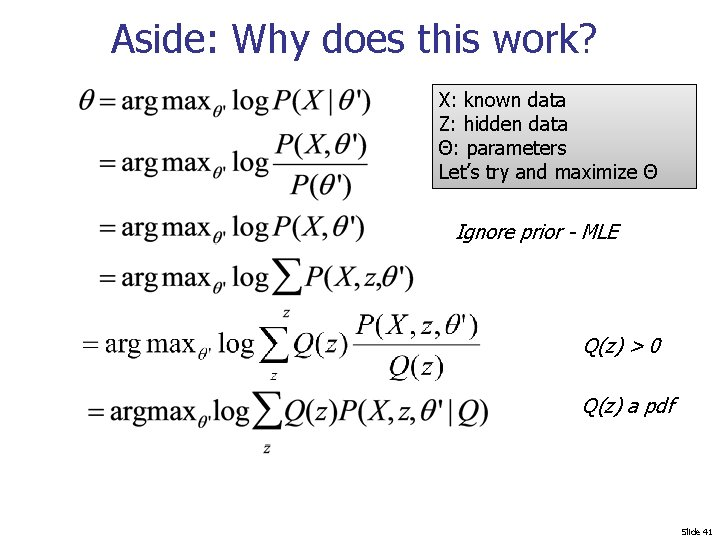Aside: Why does this work? X: known data Z: hidden data Θ: parameters Let’s try and maximize Θ Ignore prior - MLE Q(z) > 0 Q(z) a pdf Slide 41Aside: Why does this work? Ignore prior Q(z) a pdf Q(z) > 0 Initial estimate of θ This isn’t really right though: what we’re doing is Slide 42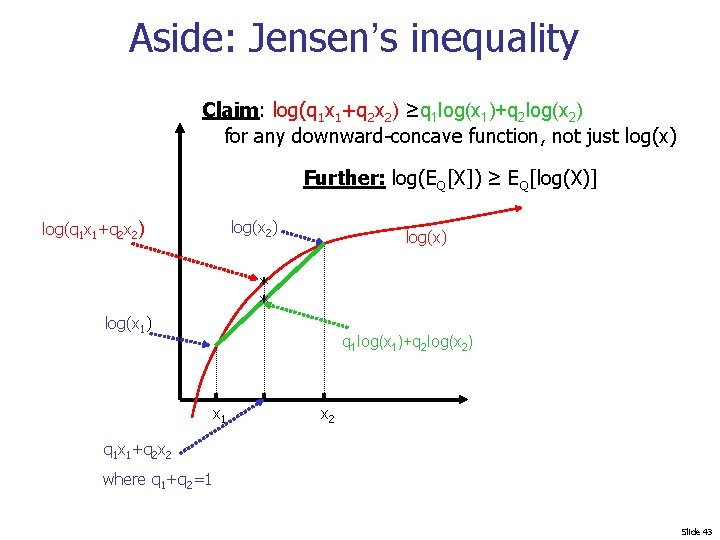Aside: Jensen’s inequality Claim: log(q 1 x 1+q 2 x 2) ≥q 1 log(x 1)+q 2 log(x 2) for any downward-concave function, not just log(x) Further: log(EQ[X]) ≥ EQ[log(X)] log(q 1 x 1+q 2 x 2) log(x) * * log(x 1) q 1 log(x 1)+q 2 log(x 2) x 1 x 2 q 1 x 1+q 2 x 2 where q 1+q 2=1 Slide 43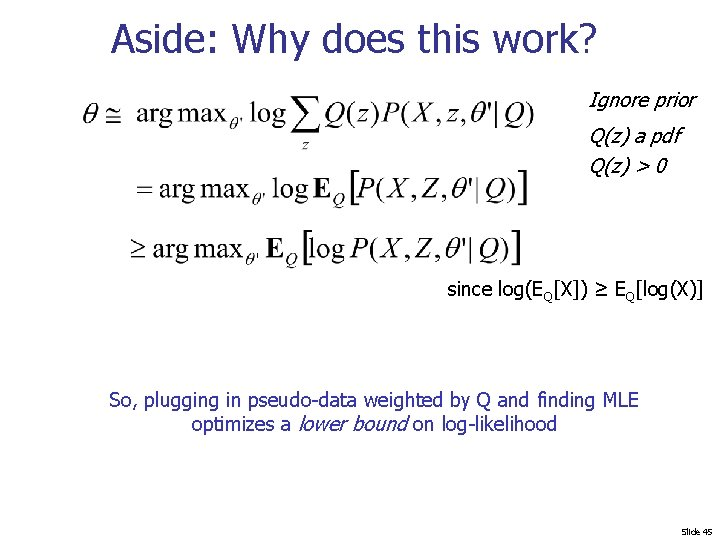Aside: Why does this work? Ignore prior Q(z) a pdf Q(z) > 0 since log(EQ[X]) ≥ EQ[log(X)] So, plugging in pseudo-data weighted by Q and finding MLE optimizes a lower bound on log-likelihood Slide 45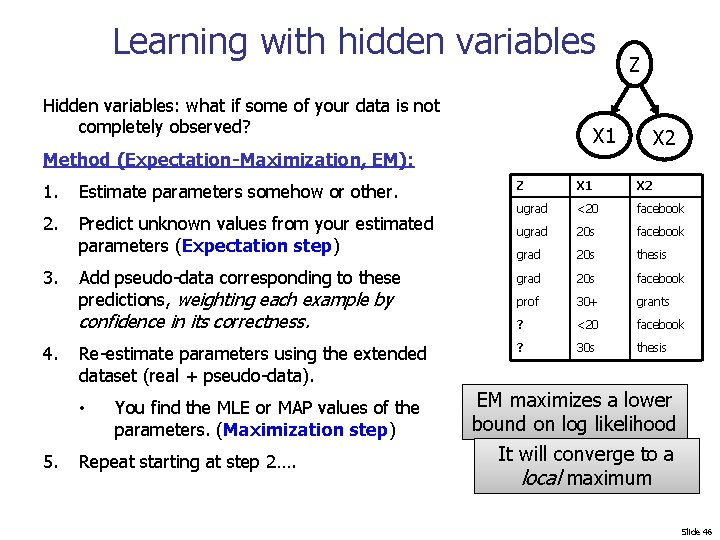Learning with hidden variables Hidden variables: what if some of your data is not completely observed? Z X 1 X 2 Method (Expectation-Maximization, EM): 1. Estimate parameters somehow or other. Z X 1 X 2 2. Predict unknown values from your estimated parameters (Expectation step) ugrad <20 facebook ugrad 20 s facebook grad 20 s thesis grad 20 s facebook prof 30+ grants ? <20 facebook ? 30 s thesis 3. Add pseudo-data corresponding to these predictions, weighting each example by confidence in its correctness. 4. Re-estimate parameters using the extended dataset (real + pseudo-data). • 5. You find the MLE or MAP values of the parameters. (Maximization step) Repeat starting at step 2…. EM maximizes a lower bound on log likelihood It will converge to a local maximum Slide 46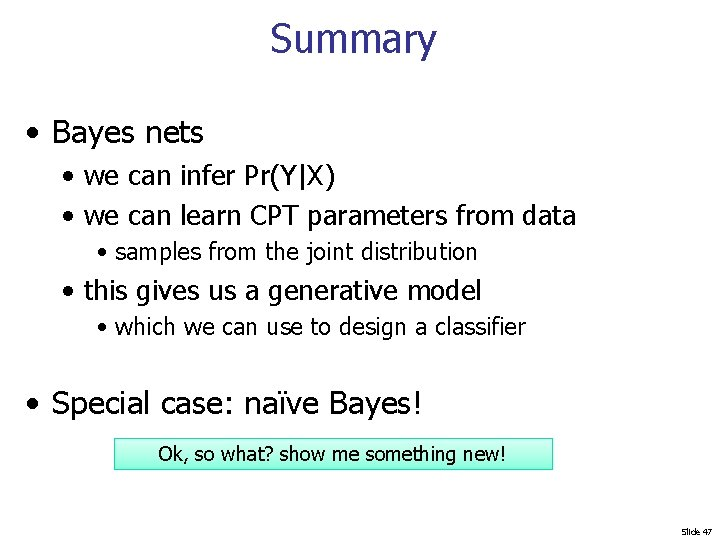Summary • Bayes nets • we can infer Pr(Y|X) • we can learn CPT parameters from data • samples from the joint distribution • this gives us a generative model • which we can use to design a classifier • Special case: naïve Bayes! Ok, so what? show me something new! Slide 47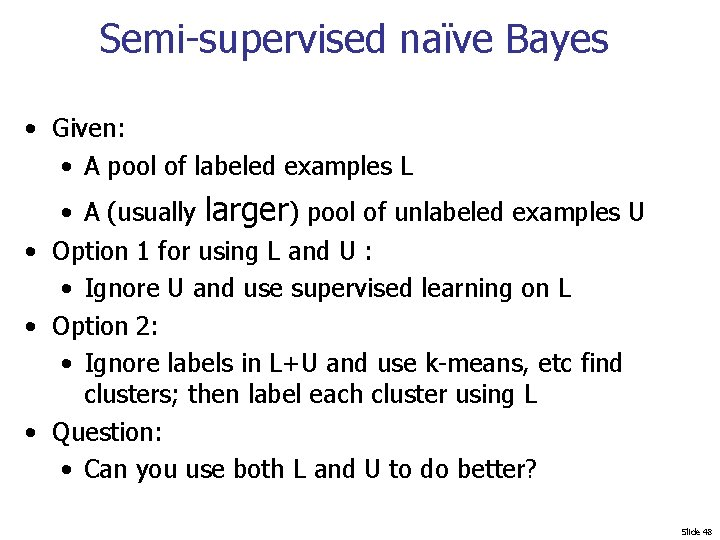Semi-supervised naïve Bayes • Given: • A pool of labeled examples L • A (usually larger) pool of unlabeled examples U • Option 1 for using L and U : • Ignore U and use supervised learning on L • Option 2: • Ignore labels in L+U and use k-means, etc find clusters; then label each cluster using L • Question: • Can you use both L and U to do better? Slide 48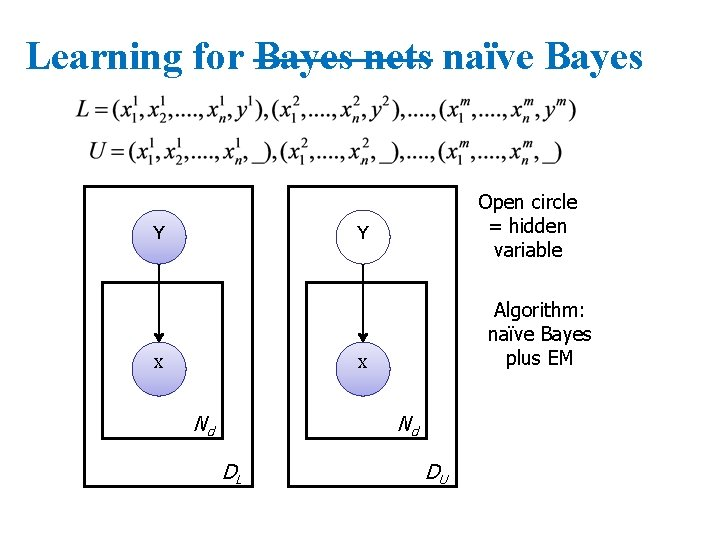Learning for Bayes nets naïve Bayes Y Open circle = hidden variable Y X Algorithm: naïve Bayes plus EM X Nd Nd DL DUSlide 50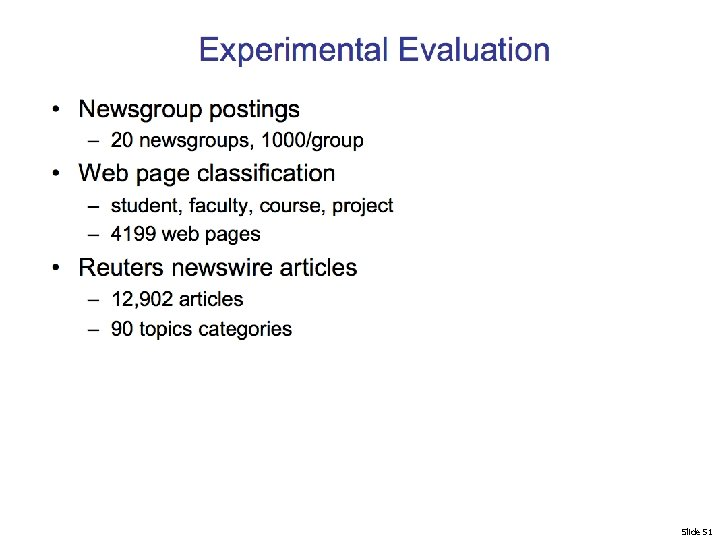Slide 51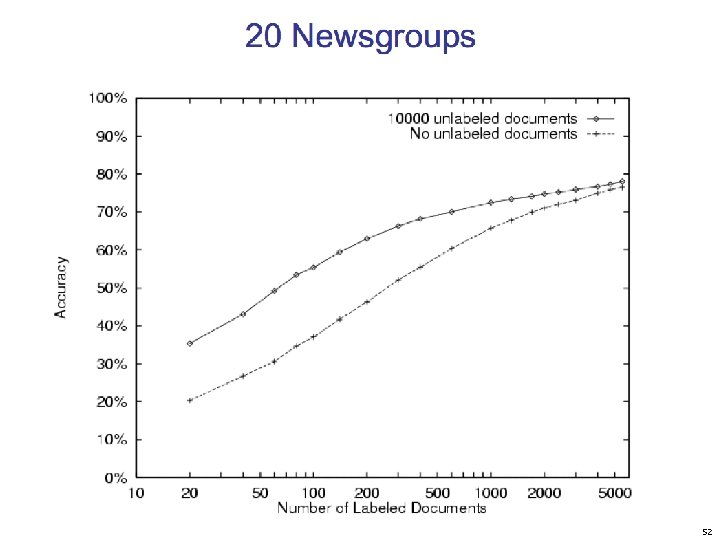Slide 52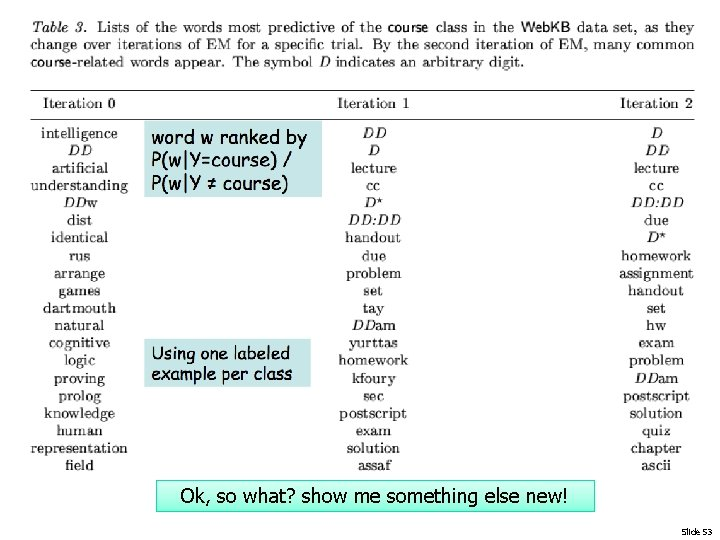Ok, so what? show me something else new! Slide 53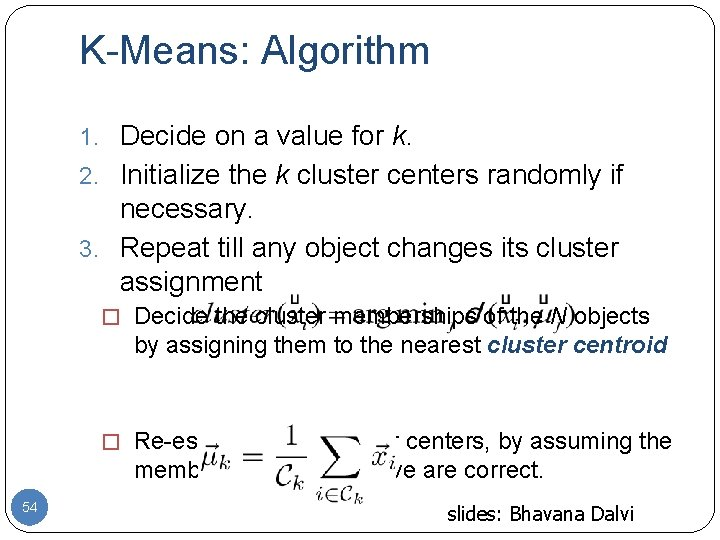K-Means: Algorithm 1. Decide on a value for k. 2. Initialize the k cluster centers randomly if necessary. 3. Repeat till any object changes its cluster assignment � Decide the cluster memberships of the N objects by assigning them to the nearest cluster centroid � Re-estimate the k cluster centers, by assuming the memberships found above are correct. 54 slides: Bhavana Dalvi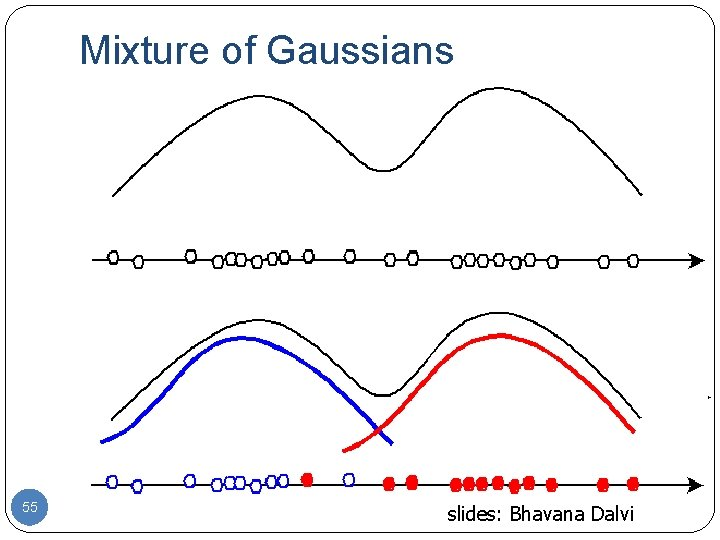Mixture of Gaussians 55 slides: Bhavana DalviA Bayes network for a mixture of Gaussians #hidden mixture component Z Pr(X|z=k) is a Gaussian, not a multinomial. X M 56 #examples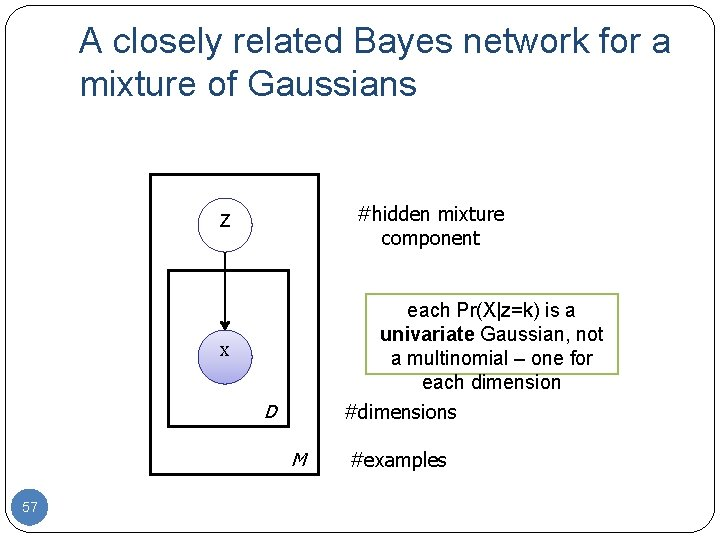A closely related Bayes network for a mixture of Gaussians #hidden mixture component Z each Pr(X|z=k) is a univariate Gaussian, not a multinomial – one for each dimension X D #dimensions M 57 #examples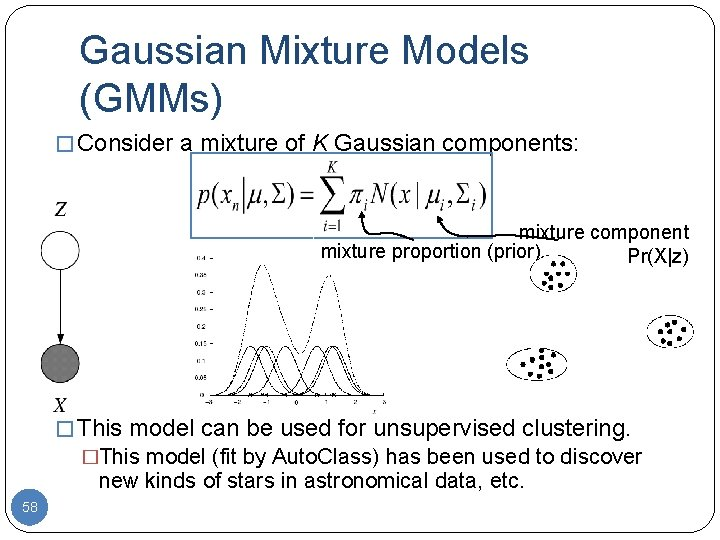Gaussian Mixture Models (GMMs) � Consider a mixture of K Gaussian components: mixture component mixture proportion (prior) Pr(X|z) � This model can be used for unsupervised clustering. �This model (fit by Auto. Class) has been used to discover new kinds of stars in astronomical data, etc. 58Expectation-Maximization (EM) �Start: "Guess" the mean and covariance of each of the K gaussians �Loop 59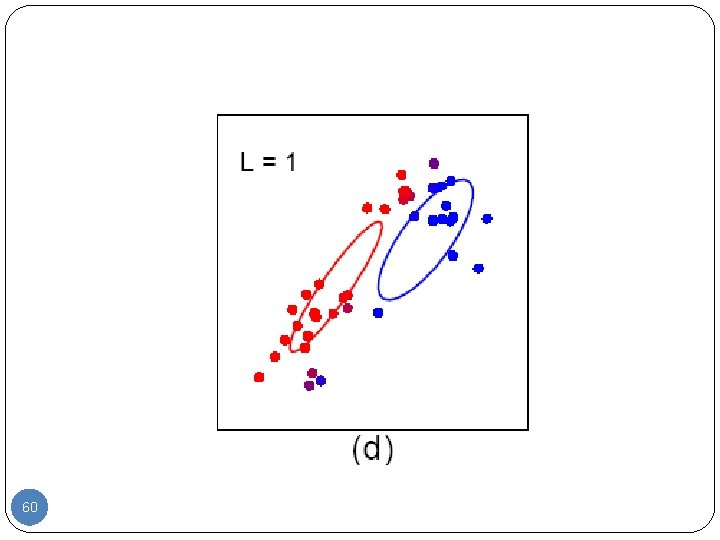60Expectation-Maximization (EM) �Start: "Guess" the centroid and covariance of each of the K clusters �Loop 61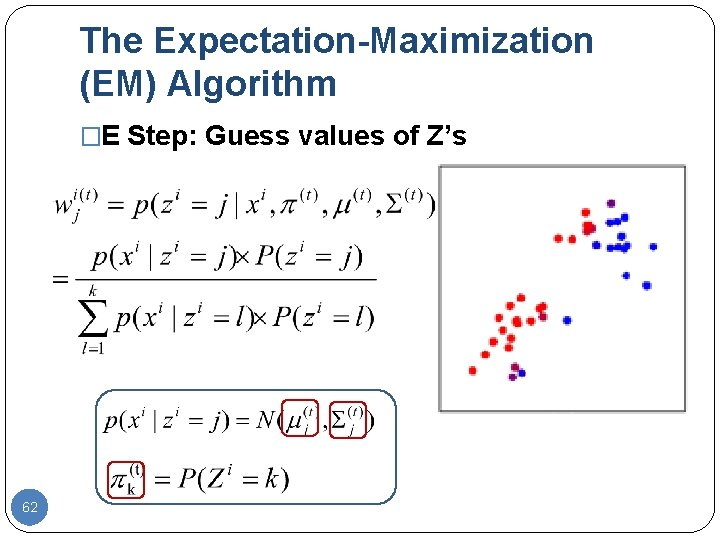The Expectation-Maximization (EM) Algorithm �E Step: Guess values of Z’s 62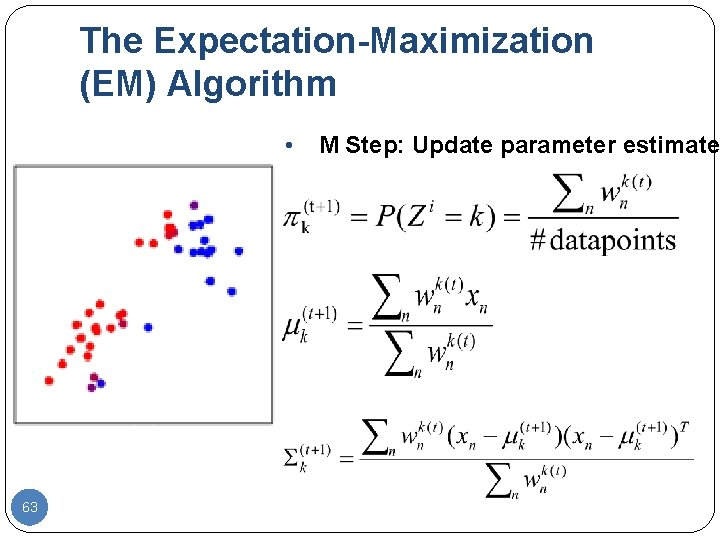The Expectation-Maximization (EM) Algorithm • 63 M Step: Update parameter estimatesEM Algorithm for GMM �E Step: Guess values of Z’s • 64 M Step: Update parameter estimatesK-means is a hard version of EM �In the K-means “E-step” we do hard assignment: �In the K-means “M-step” we update the means as the weighted sum of the data, but now the weights are 0 or 1: 65Soft vs. Hard EM assignments �GMM 66 �K-Means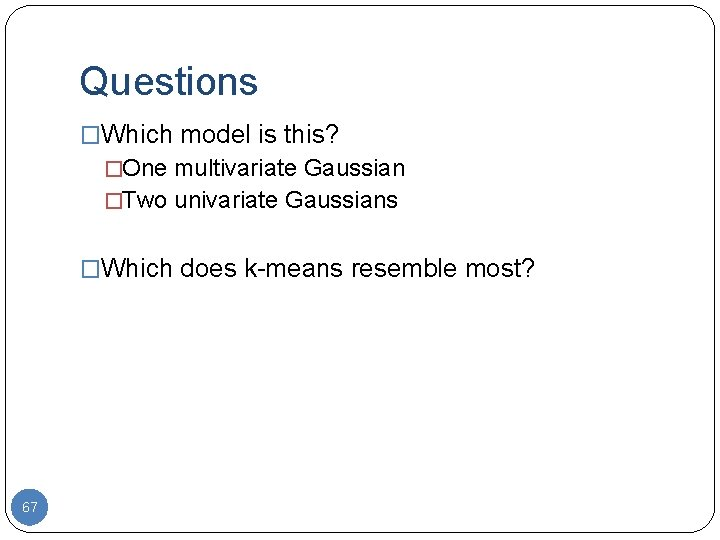Questions �Which model is this? �One multivariate Gaussian �Two univariate Gaussians �Which does k-means resemble most? 67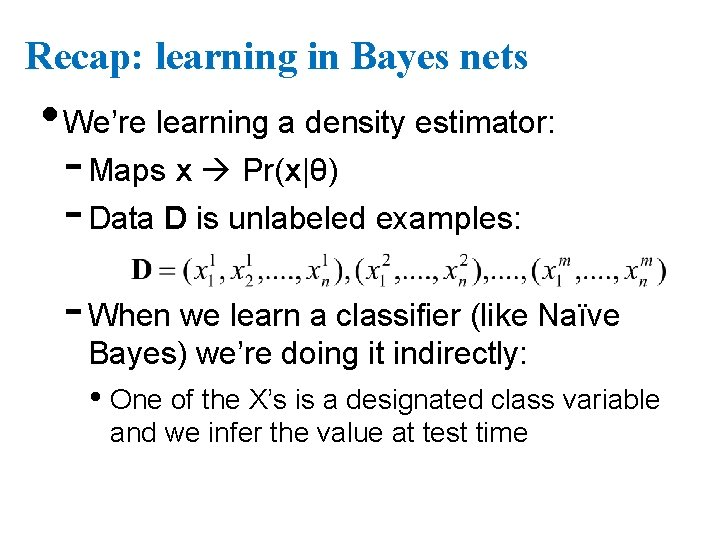Recap: learning in Bayes nets • We’re learning a density estimator: - Maps x Pr(x|θ) - Data D is unlabeled examples: - When we learn a classifier (like Naïve Bayes) we’re doing it indirectly: • One of the X’s is a designated class variable and we infer the value at test timeRecap: learning in Bayes nets • Simple case: all variables are observed - We just estimate the CPTs from the data using a MAP estimate • Harder case: some variables are hidden • We can use EM: - Repeatedly find θ, Z, … - Find expectations over Z with inference - Maximize θ|Z by with MAP over pseudo-data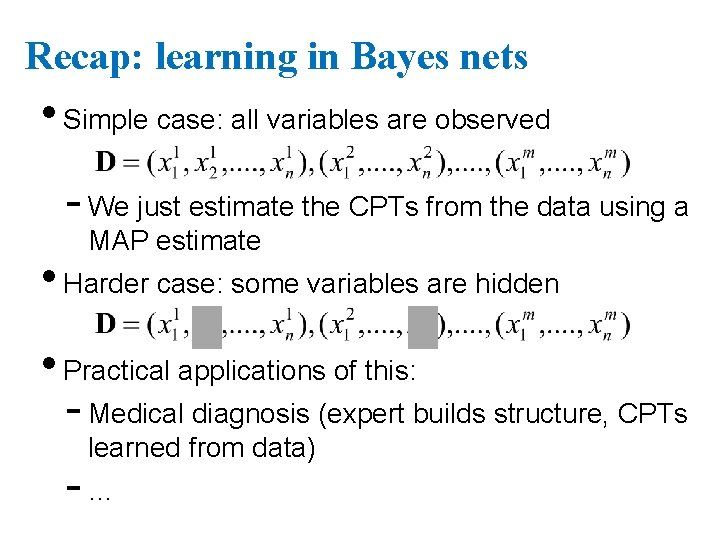Recap: learning in Bayes nets • Simple case: all variables are observed - We just estimate the CPTs from the data using a MAP estimate • Harder case: some variables are hidden • Practical applications of this: - Medical diagnosis (expert builds structure, CPTs learned from data) -…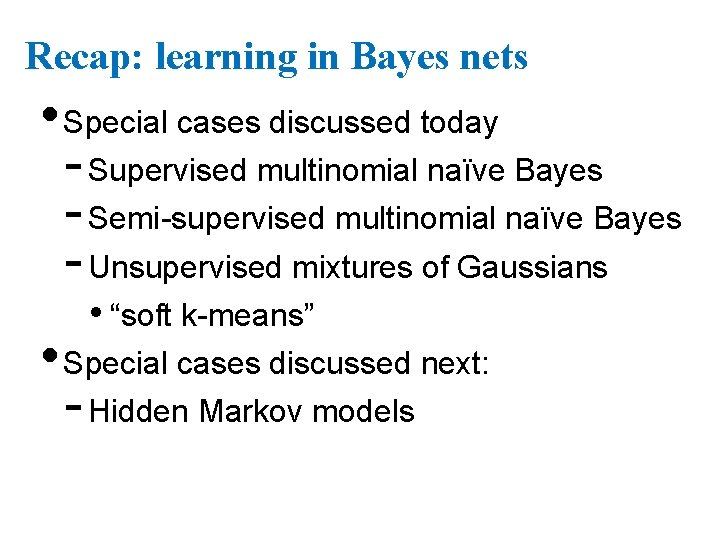Recap: learning in Bayes nets • Special cases discussed today - Supervised multinomial naïve Bayes - Semi-supervised multinomial naïve Bayes - Unsupervised mixtures of Gaussians • “soft k-means” • Special cases discussed next: - Hidden Markov modelsSome things to think about P(X|Y) Y observed Y mixed Multinomial naïve Bayes SS naive Bayes Gaussian naïve Bayes Y hidden Mixture of multinomials Mixture of Gaussians